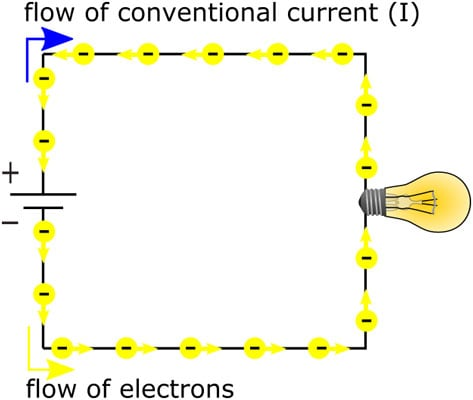##### Electronics For Dummies, 3rd EditionEarly experimenters believed that electric current was the flow of positive charges, so they described electric current as the flow of a positive charge from a positive terminal to a negative terminal. Much later, experimenters discovered electrons and determined that they flow from a negative terminal to a positive terminal.

That original convention is still around today — so the standard is to depict the direction of electric current in diagrams with an arrow that points opposite the direction of actual electron flow.

Conventional current is the flow of a positive charge from positive to negative and is the reverse of real electron flow. All descriptions of electronic circuits use conventional current, so if you see an arrow depicting current flow in a circuit diagram, you know it is showing the direction of conventional current flow. In electronics, the symbol I represents conventional current, measured in amperes (or amps, abbreviated A). You're more likely to encounter milliamps (mA) in circuits you build at home. A milliamp is one one-thousandth of an amp.Conventional current flows one way; electrons flow the other way.

In AC circuits, current is constantly reversing direction. So how do you show current flow in a circuit diagram? Which way should the arrow point? The answer is that it doesn't matter. You arbitrarily choose a direction for the current flow (known as the reference direction), and you label that current I. The value of I fluctuates up and down as the current alternates. If the value of I is negative, that just means that the (conventional) current is flowing in the direction opposite to the way the arrow is pointing.